/CSS

# <timing-function>

The `<single-transition-timing-function>` CSS data type denotes a mathematical function that describes how fast one-dimensional values change during animations. This lets you vary the animation's speed over the course of its duration.

"Smooth" timing functions are often called easing functions. They correlate a time ratio to an output ratio, both expressed as `<number>`s. For these values, `0.0` represents the initial state, and `1.0` represents the final state.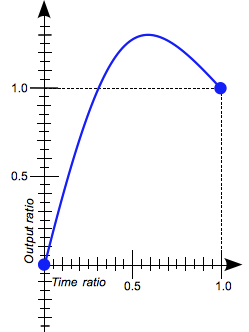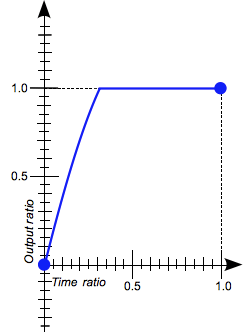Depending on the specific function used, the calculated output can sometimes grow to be greater than `1.0` or smaller than `0.0` during the course of an animation. This causes the animation to go farther than the final state, and then return. For some properties, such as `left` or `right`, this creates a kind of "bouncing" effect.

However, certain properties will restrict the output if it goes outside an allowable range. For example, a color component greater than `255` or smaller than `0` will be clipped to the closest allowed value (`255` and `0`, respectively). Some `cubic-bezier()` curves exhibit this property.

## Timing functions

CSS supports two kinds of timing functions: the subset of the cubic Bézier curves that are functions, and staircase functions. The most useful of these functions are given a keyword that allows them to be easily referenced.

### The cubic-bezier() class of timing functions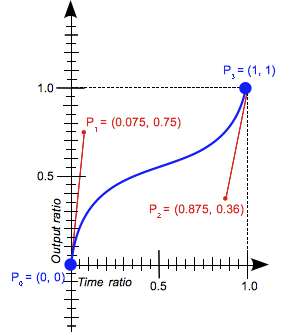The `cubic-bezier()` functional notation defines a cubic Bézier curve. As these curves are continuous, they are often used to smooth down the start and end of the animation and are therefore sometimes called easing functions.

A cubic Bézier curve is defined by four points P0, P1, P2, and P3. P0 and P3 are the start and the end of the curve and, in CSS these points are fixed as the coordinates are ratios (the abscissa the ratio of time, the ordinate the ratio of the output range). P0 is `(0, 0)` and represents the initial time and the initial state, P3 is `(1, 1)` and represents the final time and the final state.

Not all cubic Bézier curves are suitable as timing functions as not all are mathematical functions; i.e., curves that for a given abscissa have zero or one value. With P0 and P3 fixed as defined by CSS, a cubic Bézier curve is a function, and is therefore valid, if and only if the abscissas of P1 and P2 are both in the `[0, 1]` range.

Cubic Bézier curves with the P1 or P2 ordinate outside the `[0, 1]` range may generate bouncing effects.

When you specify an invalid `cubic-bezier` curve, CSS ignores the whole property.

#### Syntax

```cubic-bezier(x1, y1, x2, y2)
```

where:

x1, y1, x2, y2
Are `<number>` values representing the abscissas, and ordinates of the P1 and P2 points defining the cubic Bézier curve. x1 and x2 must be in the range [0, 1] or the value is invalid.

#### Examples

These cubic Bézier curves are valid for use in CSS:

```/* The canonical Bézier curve with four <number> in the [0,1] range. */
cubic-bezier(0.1, 0.7, 1.0, 0.1)

/* Using <integer> is valid as any <integer> is also a <number>. */
cubic-bezier(0, 0, 1, 1)

/* Negative values for ordinates are valid, leading to bouncing effects.*/
cubic-bezier(0.1, -0.6, 0.2, 0)

/* Values > 1.0 for ordinates are also valid. */
cubic-bezier(0, 1.1, 0.8, 4)
```

These cubic Bézier curves definitions are invalid:

```/* Though the animated output type may be a color,
Bézier curves work w/ numerical ratios.*/
cubic-bezier(0.1, red, 1.0, green)

/* Abscissas must be in the [0, 1] range or
the curve is not a function of time. */
cubic-bezier(2.45, 0.6, 4, 0.1)

/* The two points must be defined, there is no default value. */
cubic-bezier(0.3, 2.1)

/* Abscissas must be in the [0, 1] range or
the curve is not a function of time. */
cubic-bezier(-1.9, 0.3, -0.2, 2.1)
```

### The steps() class of timing functions

The `steps()` functional notation defines a step function dividing the domain of output values in equidistant steps.

This subclass of step functions are sometimes also called staircase functions.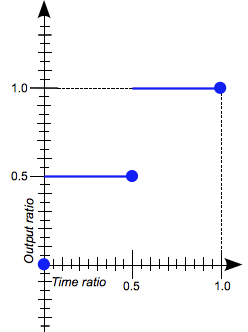`steps(2, start)`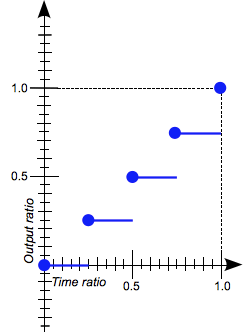`steps(4, end)`

#### Syntax

```steps(number_of_steps, direction)
```

where:

number_of_steps
Is a strictly positive `<integer>`, representing the amount of equidistant treads composing the stepping function.
direction
Is a keyword indicating if it the function is left- or right-continuous:
• `start` denotes a left-continuous function, so that the first step happens when the animation begins;
• `end` denotes a right-continuous function, so that the last step happens when the animation ends.
`end` is the default.

#### Examples

These timing functions are valid:

```/* There is 5 treads, the last one happens
right before the end of the animation. */
steps(5, end)

/* A two-step staircase, the first one happening
at the start of the animation. */
steps(2, start)

/* The second parameter is optional. */
steps(2)
```

These timing function are invalid:

```/* The first parameter must be an <integer> and
cannot be a real value, even if it is equal to one. */
steps(2.0, end)

/* The amount of steps must be non-negative. */
steps(-3, start)

/* There must be at least one step.*/
steps(0, end)```

### The frames() class of timing functions

Note: The name of the `frames()` timing function is currently under discussion, so it is currently disabled in browser release versions until a final decision is reached.

The `frames()` functional notation defines a frames function dividing the domain of output values into equidistant intervals. The difference between `frames()` and `steps()` is that with `frames()`, the start (0%) and end (100%) states are shown for an equal amount of time to the other intervals.`frames(2), frames(4)`

#### Syntax

```frames(number_of_frames)
```

where:

number_of_frames
Is a strictly positive `<integer>`, representing the amount of equidistant intervals composing the stepping function.

#### Examples

These timing functions are valid:

```/* The parameter is a positive integer. */
frames(10)
```

Note: You can see a working transition example using the frames() function in our GitHub.

These timing function are invalid:

```/* The parameter must be an <integer> and
cannot be a real value, even if it is equal to one. */
frames(2.0)

/* The amount of frames must be non-negative. */
frames(-3)

/* There must be at least two frames.*/
frames(0)
```

### Keywords for common timing functions

#### `linear`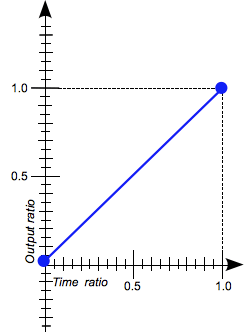The animation moves from beginning to end at a constant rate. This keyword represents the timing function `cubic-bezier(0.0, 0.0, 1.0, 1.0)`.

#### `ease`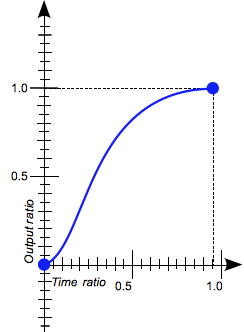The animation starts slowly, accelerates sharply, and then slows gradually towards the end. This keyword represents the timing function `cubic-bezier(0.25, 0.1, 0.25, 1.0)`. It is similar to `ease-in-out`, though it accelerates more sharply at the beginning.

#### `ease-in`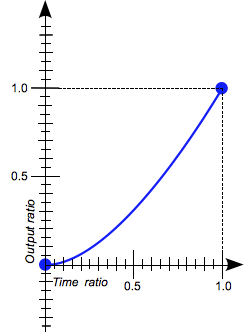The animation starts slowly, and then progressively speeds up until the end, at which point it stops abruptly. This keyword represents the timing function `cubic-bezier(0.42, 0.0, 1.0, 1.0)`.

#### `ease-in-out`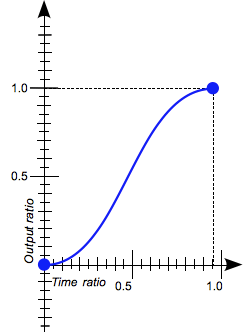The animation starts slowly, speeds up, and then slows down towards the end. This keyword represents the timing function `cubic-bezier(0.42, 0.0, 0.58, 1.0)`. At the beginning, it behaves like the `ease-in` function; at the end, it is like the `ease-out` function.

#### `ease-out`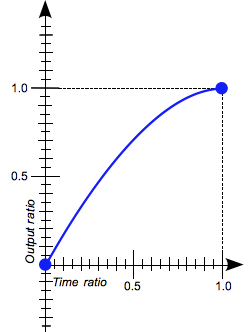The animation starts abruptly, and then progressively slows down towards the end. This keyword represents the timing function `cubic-bezier(``0.0, 0.0, 0.58, 1.0``)`.

#### `step-start`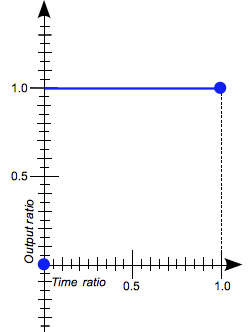The animation jumps immediately to its final state, where it stays until the end. This keyword represents the timing function `steps(1, start)`.

#### `step-end`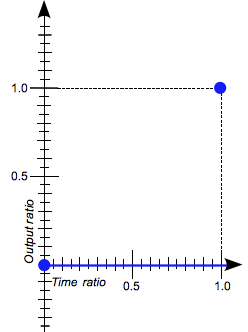The animation stays in its initial state until the end, at which point it jumps directly to its final state. This keyword represents the timing function `steps(1, end)`.

## Specifications

Specification Status Comment
CSS Animations
The definition of '<single-transition-timing-function>' in that specification.
Working Draft Defines `<single-timing-function>` as a synonym for `<single-transition-timing-function>` of the CSS Transitions Module.
CSS Transitions
The definition of '<single-transition-timing-function>' in that specification.
Working Draft Initial definition.

## Browser compatibilityUpdate compatibility data on GitHub

Desktop
Chrome Edge Firefox Internet Explorer Opera Safari
Basic support 4 ? 4 10 10.5 3.1
`cubic-bezier()` with ordinate ∉ [0,1] 16 ? 4 10 12.1 Yes
`steps()` 8 ? 4 10 12.1 5.1
Mobile
Android webview Chrome for Android Edge Mobile Firefox for Android Opera for Android iOS Safari Samsung Internet
Basic support 4 ? ? 4 10 2 ?
`cubic-bezier()` with ordinate ∉ [0,1] Yes ? ? 4 ? ? ?
`steps()` 4 ? ? 4 ? 5 ?